# Last wave problem ever

## Homework Statement

The question is to sketch for values of t = 0, t =0.25, t = 1, t=1.25 and t = 1.5 for the wave equation

Ytt = Yxx in the semi-infinite domain with initial conditions

y(x,0) = f(x) =
\begin{cases}
1 & \text{if } 0≤x≤1 \\
0 & otherwise
\end{cases}

Yt (x,0) = 0 and boundary condition of y(0,t) = 0

so I changed f(x) into D(x) where

D(x) =
\begin{cases}
1 & \text{if } 0≤x≤1 \\
-1 & \text{if } -1≤x≤0 \\
0 & otherwise
\end{cases}

## Homework Equations

Y(x,t) = Φa(x,t) +Φb(x,t)

where Φa(x,t) = 1/2 D(x+t)
and Φb(x,t) = 1/2 D(x-t)

## The Attempt at a Solution

These are the sketches. Can somebody please tell me if they are all correct for this question!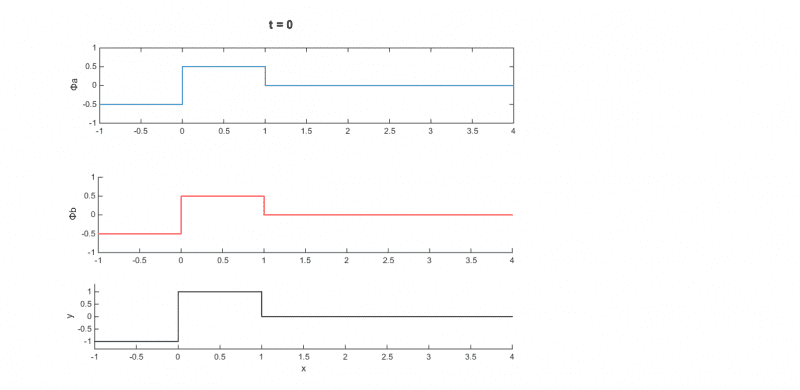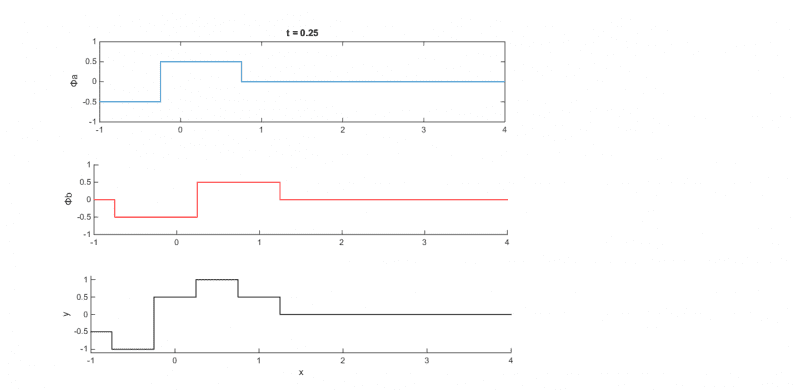Orodruin
Staff Emeritus
Homework Helper
Gold Member
2021 Award
The decomposition into φa and φb looks correct and they seem to travel in the correct direction. However, the bottom result for y is not correct. You can see this easily as it does not fulfil the boundary condition, i.e., it has y(0) = 0.5.

The decomposition into φa and φb looks correct and they seem to travel in the correct direction. However, the bottom result for y is not correct. You can see this easily as it does not fulfil the boundary condition, i.e., it has y(0) = 0.5.

Both of the y seems to have problem...do you have any idea how can I fix this? Because If I want to make y 0 whenever x is 0 then my two parts diagram will be affected right? I thought both a and b parts are correct in the diagram so I don't want to change them...

LCKurtz
Homework Helper
Gold Member
Your graphs would be much easier to interpret if you would plot both waves and their sum in the same picture. Also show your x axis passing through y = 0 instead of under the graph, and finally, use a symmetric range like x = -4 to 4.

Chestermiller
Mentor
At t = 0.25, the red and the blue plots are correct. They just don't add up to the black curve as you have drawn it.

Chet

At t = 0.25, the red and the blue plots are correct. They just don't add up to the black curve as you have drawn it.

Chet

how does it add up then? I mean I just went straight up at x = -0.25 cause the blue part starts there...or do I just have to make y =0 at x =0 then continue as normal? Also can you confirm if t =0 is correct?

Chestermiller
Mentor
how does it add up then? I mean I just went straight up at x = -0.25 cause the blue part starts there...or do I just have to make y =0 at x =0 then continue as normal?
No. You added the curves up incorrectly between -0.25 and +0.25. Otherwise, the rest is OK
Also can you confirm if t =0 is correct?
Confirmed.

Chet

No. You added the curves up incorrectly between -0.25 and +0.25. Otherwise, the rest is OK

Confirmed.

Chet

So the boundary condition is satisfied in t = 0? But how though when x is 0 isn't the y 1? shouldn't it be 0?

Also for this question when t = 1 is this correct?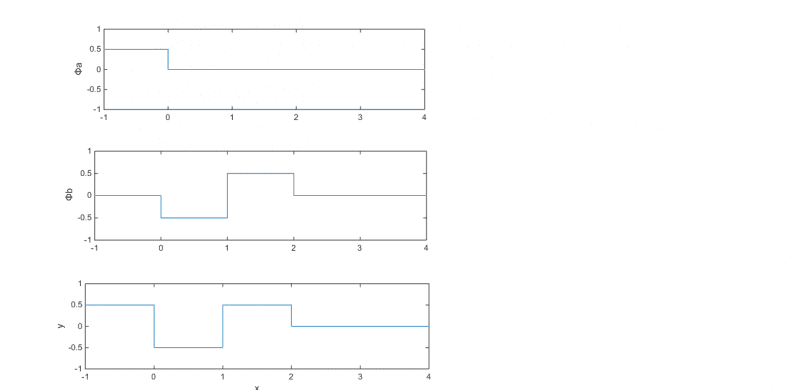Chestermiller
Mentor
So the boundary condition is satisfied in t = 0? But how though when x is 0 isn't the y 1? shouldn't it be 0?
I don't understand this question grammatically.

Chet

I mean when t=0 how is the boundary condition being satisfied?

Also can you confirm if sketch for t=1 is correct

Chestermiller
Mentor
I mean when t=0 how is the boundary condition being satisfied?
At each of the jumps, the value exactly at the jump is the average of the values on either side.
Also can you confirm if sketch for t=1 is correct
No. The middle sketch is not correct. Anyway, you should only be looking at the region x > 0 (for the combined solution).

Chet

At each of the jumps, the value exactly at the jump is the average of the values on either side.

No. The middle sketch is not correct. Anyway, you should only be looking at the region x > 0 (for the combined solution).

Chet

For t=0.25 is this sketch for y correct then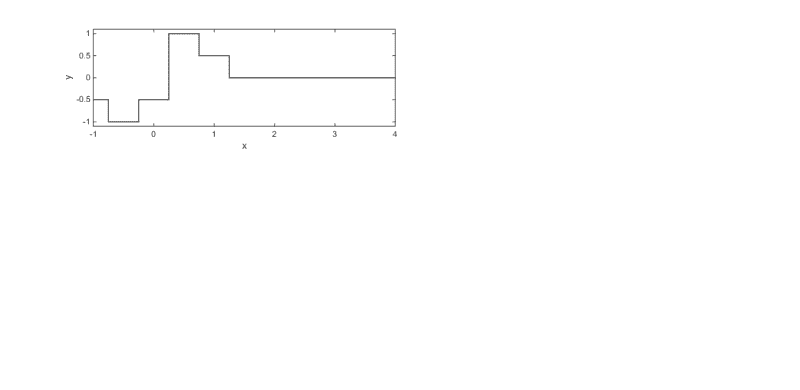and for t = 1 by middle sketch you mean the Φb is not correct and other two are correct? and as for considering x>0 for combined solution, that's what I have done for the y sketch of t = 1....I have ignored the x<0 region of the Φb sketch...but added the x>0 region of Φa and Φb

Chestermiller
Mentor
For t=0.25 is this sketch for y correct then

View attachment 77413
No. y should be zero between x = -0.25 and x = + 0.25.
and for t = 1 by middle sketch you mean the Φb is not correct and other two are correct?
No. Both the middle sketch and the bottom sketch are wrong.

and as for considering x>0 for combined solution, that's what I have done for the y sketch of t = 1....I have ignored the x<0 region of the Φb sketch...but added the x>0 region of Φa and Φb
Then just show that region so we're not confused. But, if you do show the region x < 0, then you should show the correct sketches there.

Chet

No. y should be zero between x = -0.25 and x = + 0.25.

No. Both the middle sketch and the bottom sketch are wrong.

Then just show that region so we're not confused. But, if you do show the region x < 0, then you should show the correct sketches there.

Chet

What am I doing wrong for the middle and the bottom sketches? I mean for the middle part specially I am considering the range D(x) = -1 if -1<x<0 so as t = 1 i am adding -1+1<x<0+1

so my range becomes 0<x<1 for Φb = -0.5

and when considering D(x) = 1 if 0<x<1 for t = 1 I am adding again so 0+1<x<1+1 so my range becomes 1<x<2 for Φb = 0.5

What's wrong in here....I don't understand

Chestermiller
Mentor
What am I doing wrong for the middle and the bottom sketches? I mean for the middle part specially I am considering the range D(x) = -1 if -1<x<0 so as t = 1 i am adding -1+1<x<0+1

so my range becomes 0<x<1 for Φb = -0.5

and when considering D(x) = 1 if 0<x<1 for t = 1 I am adding again so 0+1<x<1+1 so my range becomes 1<x<2 for Φb = 0.5

What's wrong in here....I don't understand
Like I said, if you are going to show the region x <0 on the sketch, it should be shown correctly. In the region between x = -1 and x = 0, the middle sketch should be -0.5.

Chet

Like I said, if you are going to show the region x <0 on the sketch, it should be shown correctly. In the region between x = -1 and x = 0, the middle sketch should be -0.5.

Chet

Is this correct for t =0.25 y sketch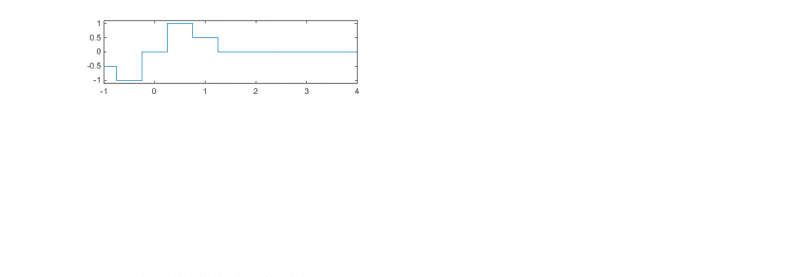Like I said, if you are going to show the region x <0 on the sketch, it should be shown correctly. In the region between x = -1 and x = 0, the middle sketch should be -0.5.

Chet

And for t=1 are the middle and bottom part correct now?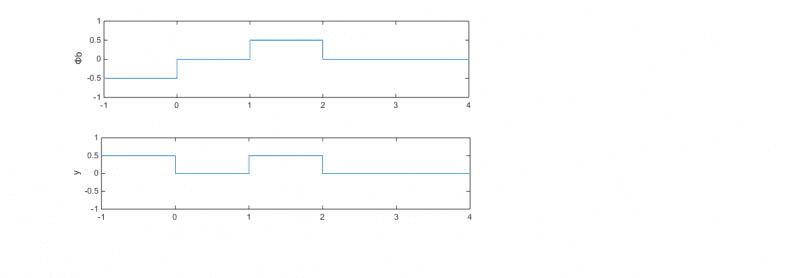Chestermiller
Mentor
And for t=1 are the middle and bottom part correct now?

View attachment 77417
No. Both are wrong. On the middle sketch, it should be -0.5 from x = 0 to x = 1, and 0 from x = -1 to x = 0.

Chet

I don't understand this question grammatically.

Chet

Like I said, if you are going to show the region x <0 on the sketch, it should be shown correctly. In the region between x = -1 and x = 0, the middle sketch should be -0.5.

Chet

No. Both are wrong. On the middle sketch, it should be -0.5 from x = 0 to x = 1, and 0 from x = -1 to x = 0.

Chet

In that case my first middle sketch for t=1 is correct then if you have a look. I do have -0.5 for 0 to 1 and 0 for -1 to 0. Why did you say it was wrong initially then?

Chestermiller
Mentor
In that case my first middle sketch for t=1 is correct then if you have a look. I do have -0.5 for 0 to 1 and 0 for -1 to 0. Why did you say it was wrong initially then?
Ooops. My Mistake. Sorry.

Chet

Ooops. My Mistake. Sorry.

Chet

That's alright...can you check the latest t =0.25 I posted in post 17...is that correct sketch for y?

Chestermiller
Mentor
That's alright...can you check the latest t =0.25 I posted in post 17...is that correct sketch for y?
Yes.